THEORETICAL PROBLEMS IN BINOMIAL THEOREM

1. By substituting an appropriate value for x, prove the following identities:

(a)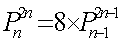(b)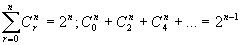(c)(d)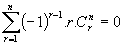(e)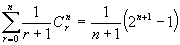(f)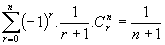(g)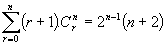(h)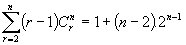2. By considering the expansion of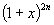in ascending powers of x, prove the following identities:

(a)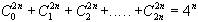(b)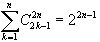(c)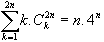and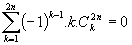(Hint: differentiate both sides of the expansion)

(d)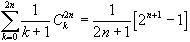and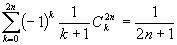(Hint: integrate both sides of the expansion)

3. If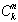is the coefficient of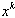in the expansion of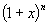, where n is a positive integer, show by differentiating the identity

(a)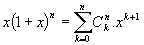that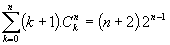(b)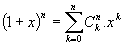that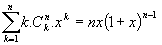(c)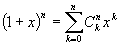TWICE that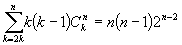4. Write down the value of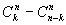. By comparing the coefficients of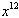on both sides of the identity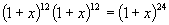, show that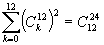. (ans: value 0).

5. From the identity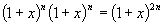, by considering the coefficient of

(i)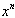on both sides, show that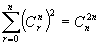(ii)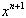on both sides, prove that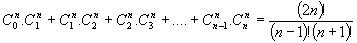.

6. By equating the coefficient of xr on each side of the identities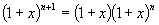and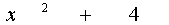, prove that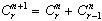and that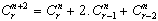.

7. By considering the coefficients of xn on both sides of the identity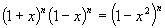, prove that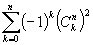is equal to

(a) 0 if n is odd; (b)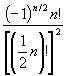if n is even.

8. (i) By considering the coefficient of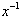on both sides of the identity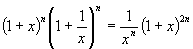, prove that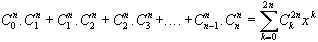(ii) If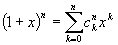and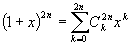, and by considering the coefficients of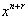in the product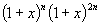to obtain the value of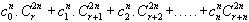(ans: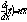)

(Hint: write the expansion of (1+x)n in reverse)

9. (i) By evaluating the integral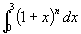in two different ways, prove the identity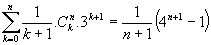(ii) Express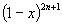in sigma notation. Then by eveluating the integral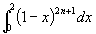in two different ways, prove the identity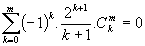where m = 2n+1. (ans: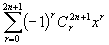)

10. Find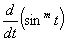and hence evaluate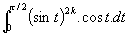(i) Noting that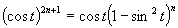and by using the binomial theorem to expand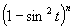where n is a positive integer, prove that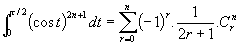.

(ii) Use the above result to find the value (rational number) of the integral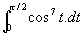.

(ans: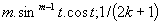(ii)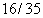).